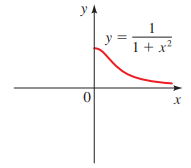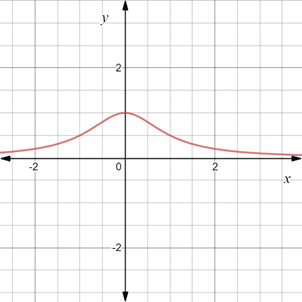# To graph : The equation y = 1 1 + x 2 such that it is symmetric with respect to y-axis.### Precalculus: Mathematics for Calcu...

6th Edition
Stewart + 5 others
Publisher: Cengage Learning
ISBN: 9780840068071### Precalculus: Mathematics for Calcu...

6th Edition
Stewart + 5 others
Publisher: Cengage Learning
ISBN: 9780840068071

#### Solutions

Chapter 1.8, Problem 83E
To determine

## To graph: The equation y=11+x2 such that it is symmetric with respect to y-axis.Expert Solution

### Explanation of Solution

Given information:

The graph of the equation y=11+x2 .Graph:

The graph of the equation y=11+x2 such that it is symmetric with respect to y-axis is provided below,Interpretation:

Graph of the equation is symmetric with respect to y-axis means that graph is not changed when reflected about the y-axis.

The equation is symmetric about the y-axis, when x is replaced by x , the equation remains unchanged.

### Have a homework question?

Subscribe to bartleby learn! Ask subject matter experts 30 homework questions each month. Plus, you’ll have access to millions of step-by-step textbook answers!